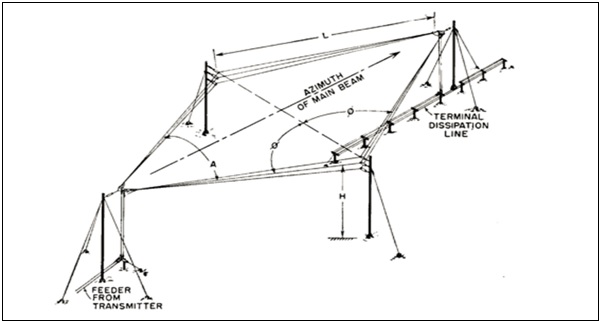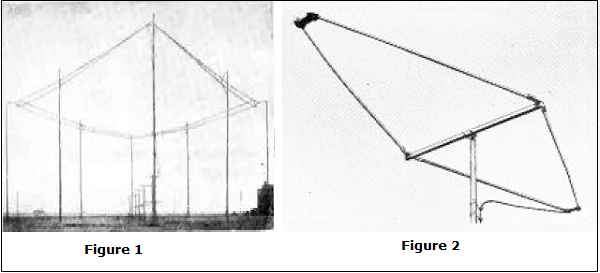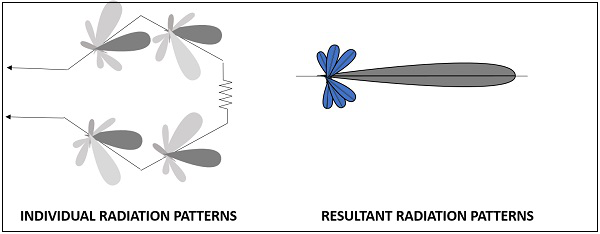# Antenna Theory - Rhombic

The Rhombic Antenna is an equilateral parallelogram shaped antenna. Generally, it has two opposite acute angles. The tilt angle, θ is approximately equal to 90° minus the angle of major lobe. Rhombic antenna works under the principle of travelling wave radiator. It is arranged in the form of a rhombus or diamond shape and suspended horizontally above the surface of the earth.

### Frequency Range

The frequency range of operation of a Rhombic antenna is around 3MHz to 300MHz. This antenna works in HF and VHF ranges.

## Construction of Rhombic Antenna

Rhombic antenna can be regarded as two V-shaped antennas connected end-to-end to form obtuse angles. Due to its simplicity and ease of construction, it has many uses −

• In HF transmission and reception

• Commercial point-to-point communication

The construction of the rhombic antenna is in the form a rhombus, as shown in the figure.The two sides of rhombus are considered as the conductors of a two-wire transmission line. When this system is properly designed, there is a concentration of radiation along the main axis of radiation. In practice, half of the power is dissipated in the terminating resistance of the antenna. The rest of the power is radiated. The wasted power contributes to the minor lobes.Figure 1 shows the construction of rhombic antenna for point-to-point communication in olden days. Figure 2 shows the rhombic UHF antenna for TV reception, used these days.

The maximum gain from a rhombic antenna is along the direction of the main axis, which passes through the feed point to terminate in free space. The polarization obtained from a horizontal rhombic antenna is in the plane of rhombus, which is horizontal.

The radiation pattern of the rhombic antenna is shown in the following figure. The resultant pattern is the cumulative effect of the radiation at all four legs of the antenna. This pattern is uni-directional, while it can be made bi-directional by removing the terminating resistance.The main disadvantage of rhombic antenna is that the portions of the radiation, which do not combine with the main lobe, result in considerable side lobes having both horizontal and vertical polarization.

The following are the advantages of Rhombic antenna −

• Input impedance and radiation pattern are relatively constant

• Multiple rhombic antennas can be connected

• Simple and effective transmission

The following are the disadvantages of Rhombic antenna −

• Wastage of power in terminating resistor

• Requirement of large space

• Redued transmission efficiency

### Applications

The following are the applications of Rhombic antenna −

• Used in HF communications

• Used in Long distance sky wave propagations

• Used in point-to-point communications

Another method of using long wire is by bending and making the wire into a loop shaped pattern and observing its radiational parameters. This type of antennas are termed as loop antennas.